library(ape)

## Warning: package 'ape' was built under R version 2.15.1

lcx <- 1  ## contour lab cex
dumpfn <- "acedump.RData"
copy_num <- c(35, 24, 6)
names(copy_num) <- c("human", "chimp", "rhesus")
source("ace.R")  ## hacked versions of curve3d, apply2d, and ace

## Loading required package: plyr

max_copy_num = 80


Set up the transition matrix:

copy_num <- factor(copy_num, levels = 1:max_copy_num)
transitions <- matrix(0, max_copy_num, max_copy_num)
transitions[(row(transitions) - col(transitions)) == -1] <- 1
transitions[(row(transitions) - col(transitions)) == 1] <- 2


Regular ace fails:

out <- ace(copy_num, mytree, type = "discrete", model = transitions)

## Error: non-finite value supplied by 'nlm'


Now we'll use the hacked version to return just the deviance function so we can play with it:

info <- ace2(copy_num, mytree, type = "discrete", model = transitions, devInfoOnly = TRUE)


See what's in there:

names(info)

##  "np" "ip" "fn"

info$np  ##  2  info$ip

##  0.1


Generate deviance surface (by brute force):

if (!exists("cc")) {
cc <- curve3d(info$fn(c(x, y)), xlim = c(0.01, 100), ylim = c(0.01, 100), log = "xy", sys3d = "none") }  Picture: with(cc, image(x, y, z, log = "xy")) with(cc, contour(x, y, z, log = "xy", add = TRUE))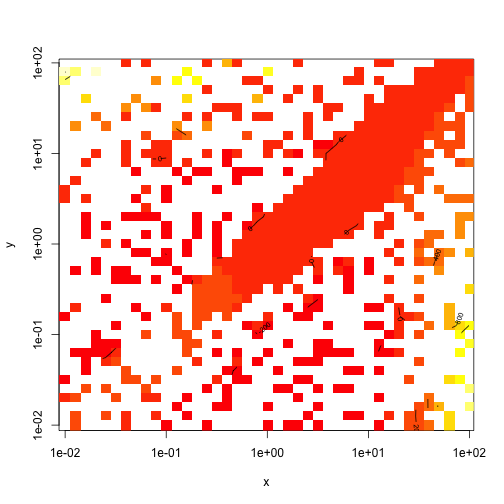Things are so horrid that the poor thing can't build a contour plot effectively. Just for comparison we'll try persp — since it can't handle infinite values we'll replace them: maxz <- with(cc, ifelse(z == Inf, max(is.finite(z)), z)) with(cc, persp(x, y, maxz))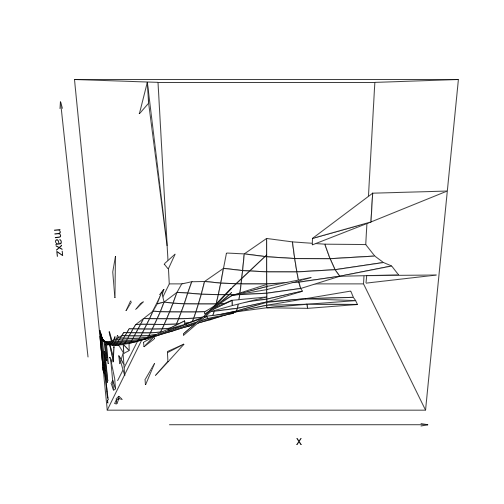Still pretty ugly! … but there are some reasonable values in the results: hist(na.omit(c(cc$z)), col = "gray", main = "")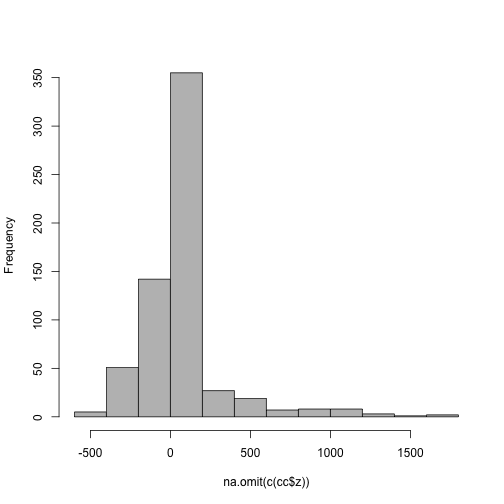Try again, over a narrower range:

if (!exists("cc2")) {
cc2 <- curve3d(info$fn(c(x, y)), xlim = c(0.1, 10), ylim = c(0.1, 10), log = "xy", sys3d = "none") }  Better, but still ugly: with(cc2, image(x, y, z, log = "xy")) with(cc2, contour(x, y, z, log = "xy", labcex = lcx, add = TRUE))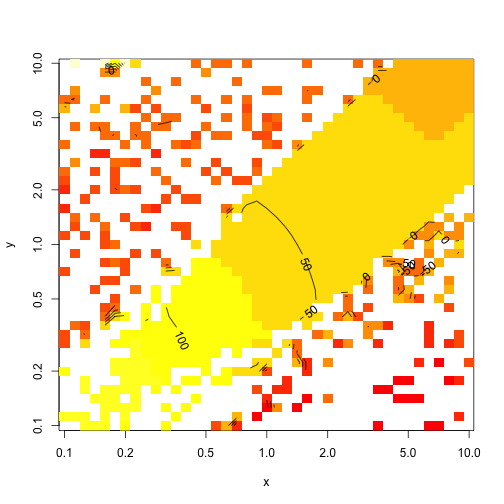If we try Nelder-Mead (a more robust algorithm), and start within the OK region, we can actually get a reasonable answer: (opt1 <- optim(fn = info$fn, par = c(1, 1), method = "Nelder-Mead"))

## $par ##  68.83 74.97 ## ##$value
##  15.23
##
## $counts ## function gradient ## 83 NA ## ##$convergence
##  0
##
## $message ## NULL  Now let's check the vicinity of those results: if (!exists("cc3")) { cc3 <- curve3d(info$fn(c(x, y)), xlim = c(50, 80), ylim = c(50, 80), sys3d = "none")
}

with(cc3, image(x, y, z))
with(cc3, contour(x, y, z, add = TRUE, labcex = lcx))
with(cc3, contour(x, y, z, add = TRUE, levels = 15:20, lty = 2, labcex = lcx))
points(opt1$par, opt1$par, pch = 16)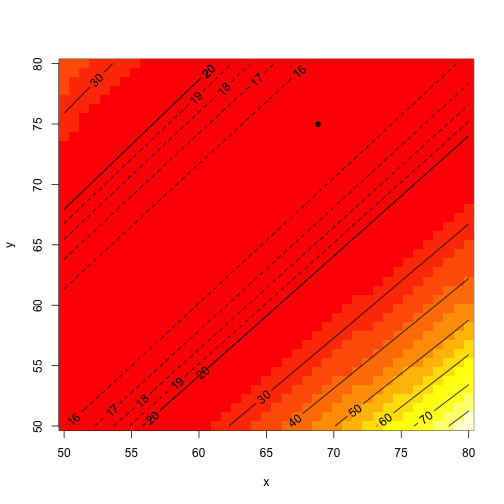If all we care about is the MLE, then we're done. I thought
we might want to know something about the confidence intervals, though \ldots
We can calculate the local curvature (Hessian) and from
that get the correlation matrix of the parameters:

library(numDeriv)
h1 <- hessian(info$fn, opt1$par)
v <- solve(h1)
cov2cor(v)

##       [,1]  [,2]
## [1,] 1.000 0.998
## [2,] 0.998 1.000


$$x$$ and $$y$$ are almost perfectly correlated.
We would be better off reparameterizing this in terms of
(either) $$x+y$$ and $$x-y$$ (I also considered $$xy$$ and $$x/y$$,
but the former seems to work OK).

fn2 <- function(sumxy, diffxy) {
x <- (sumxy + diffxy)/2
y <- sumxy - x
info$fn(c(x, y)) }  Checking: info$fn(c(1, 1))

##  56.97

fn2(2, 0)

##  56.97

info$fn(c(1, 0.7))  ##  61.52  fn2(1.7, 0.3)  ##  61.52  I drew a few surfaces, tweaking the limits outward each time: if (!exists("cc4")) { cc4 <- curve3d(fn2(x, y), xlim = c(20, 400), ylim = c(-20, 5), sys3d = "none") }  In order to fit the function on this scale we need to make a little wrapper: fn3 <- function(p) { fn2(p, p) } opt3 <- optim(fn = fn3, par = c(150, -5), method = "Nelder-Mead")  A picture: with(cc4, image(x, y, z, xlab = "x+y", ylab = "x-y", zlim = c(15, 25))) with(cc4, contour(x, y, z, add = TRUE, labcex = lcx, levels = 14:25)) with(cc4, contour(x, y, z, add = TRUE, labcex = lcx, levels = seq(15, 16, by = 0.1), lty = 2)) points(opt3$par, opt3\$par, pch = 16)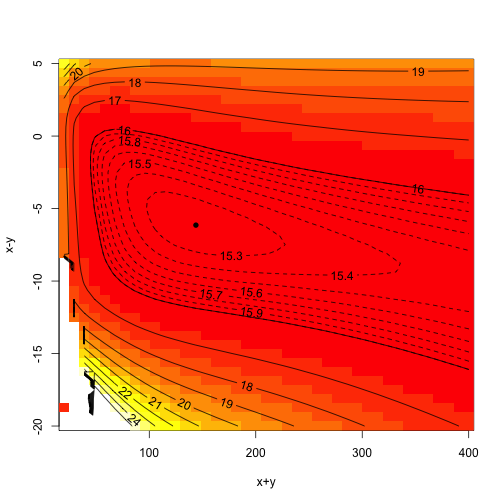Expand the range a bit more: to get 95% bivariate confidence intervals
we have to get up to (min+6) $$\approx$$ 21.3 …

if (!exists("cc5")) {
cc5 <- curve3d(fn2(x, y), xlim = c(20, 600), ylim = c(-25, 5), sys3d = "none")
}

with(cc5, image(x, y, z, xlab = "x+y", ylab = "x-y", zlim = c(15, 25)))
with(cc5, contour(x, y, z, add = TRUE, labcex = lcx, levels = 14:25))
with(cc5, contour(x, y, z, add = TRUE, labcex = lcx, levels = seq(15, 16, by = 0.1),
lty = 2))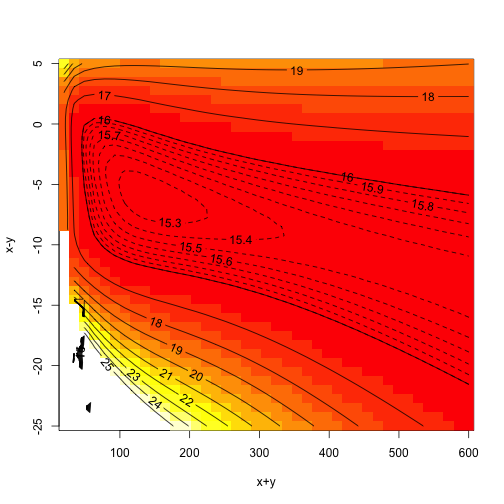We might try reparameterizing this as $$\{1/(x+y),x-y\}$$, so that we can try the boundary as $$x+y \to \infty$$?

fn2(1000, -10)  ## still not above 16!

##  15.92


Compute cross-sections of the deviance surface for a couple of large values of $$x+y$$:

xydiffvec <- seq(-200, 5, by = 1)
if (!exists("bigslice")) bigslice <- sapply(xydiffvec, function(d) fn2(5000,
d))
plot(xydiffvec, bigslice, type = "l")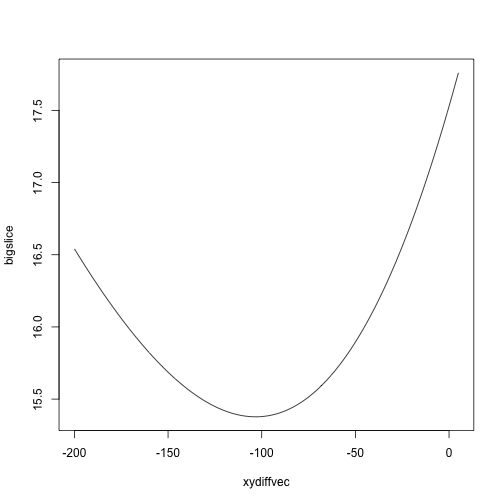The midpoint of the surface (i.e. the best value of $$x-y$$ for a given value
of $$x+y$$) keeps decreasing as we go out:

xydiffvec2 <- seq(-500, 0, by = 2)
if (!exists("bigslice2")) bigslice2 <- sapply(xydiffvec2, function(d) fn2(10000,
d))
plot(xydiffvec2, bigslice2, type = "l")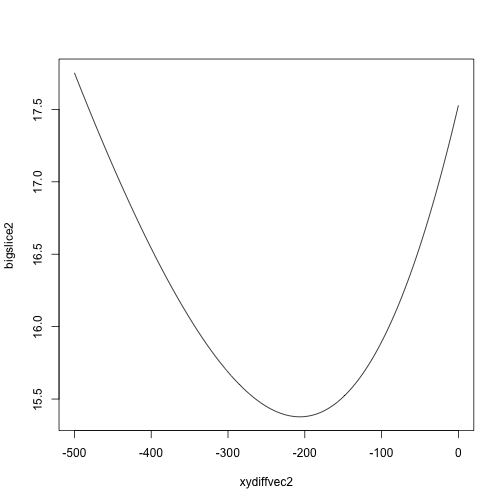Save lengthy computation results:

if (!file.exists(dumpfn)) save(list = ls(pattern = "^(cc|big)"), file = dumpfn)